# PSAT Math : Equations / Inequalities

## Example Questions

### Example Question #71 : Equations / Inequalities

Solve for: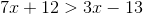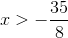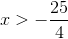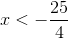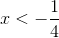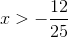Explanation:

Begin by moving all of thevalues to the left side of the inequality:becomes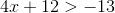Next, move theto the right side: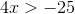Finally, divide both sides by:### Example Question #72 : Equations / Inequalities

Solve for: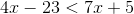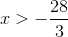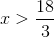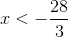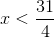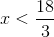Explanation:

First, move thevalues to the left side of the inequality:becomes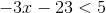Next, move the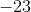to the right side: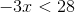Finally, divide by. Remember: you must flip the inequality sign when you multiply or divide by a negative number.### Example Question #73 : Equations / Inequalities

Solve for: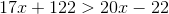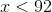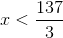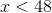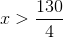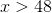Explanation:

First, get thefactors on the left side of the inequality:becomes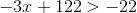Next, subtract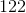from both sides: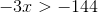Now, divide by.  Remember: Dividing or multiplying by a negative number requires you to flip the inequality sign:### Example Question #74 : Equations / Inequalities

Solve the inequality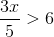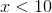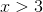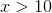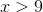Explanation:

First, multiplying each side of the equality bygives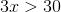. Next, dividing each side of the inequality bywill solve for.

### Example Question #1937 : Sat Mathematics

What is the solution set of the inequality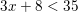?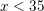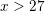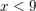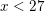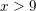Explanation:

We simplify this inequality similarly to how we would simplify an equation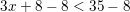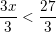Thus### Example Question #1938 : Sat Mathematics

What is a solution set of the inequality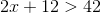?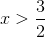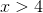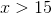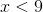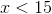Explanation:

In order to find the solution set, we solveas we would an equation: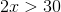Therefore, the solution set is any value of.

### Example Question #5 : How To Find Out When An Equation Has No Solution

Find the solution to the following equation if x = 3:

y = (4x2 - 2)/(9 - x2)

no possible solution

3

6

0

no possible solution

Explanation:

Substituting 3 in for x, you will get 0 in the denominator of the fraction. It is not possible to have 0 be the denominator for a fraction so there is no possible solution to this equation.

### Example Question #2 : Equations / Inequalities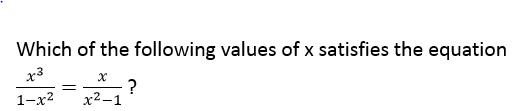I.  x = 0

II. x = –1

III. x = 1

I only

I, II, and III

II and III only

III only

II only

I only

Explanation: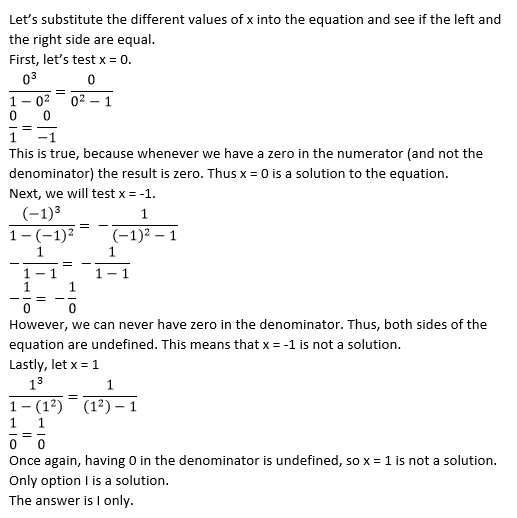### Example Question #6 : How To Find Out When An Equation Has No Solution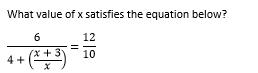–1/2

1

–3

There is no solution

3

There is no solution

Explanation: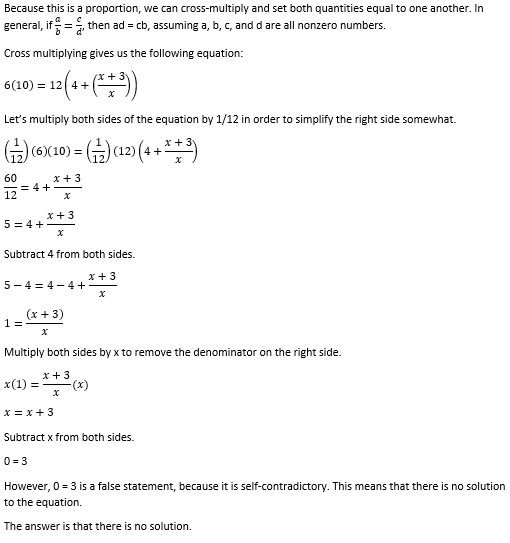### Example Question #7 : How To Find Out When An Equation Has No Solution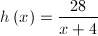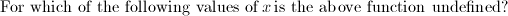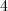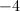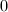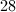Explanation:

A fraction is considered undefined when the denominator equals 0. Set the denominator equal to zero and solve for the variable.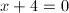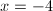### All PSAT Math Resources